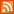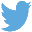Bucaro TecHelpHTTPS Encryption not required because no account numbers orpersonal information is ever requested or accepted by this siteCustom Search# JavaScript toString Method Converts a Number to a String

The JavaScript toString method is used to convert a number to a string. It accepts a single optional parameter which specifies the base in which the number is to be represented in the string. This is a very convenient method of converting a number to a different base. If called without a parameter then the digits in the number will be converted to a string directly. Shown below is code converting a number to a string without specifying a base.

```var numValue = 123;
alert("The number is " + numValue.toString());
```

The output from the above code is "The number is 123". Shown below is code passing the parameter 16 to convert the number from decimal to hexadecimal.

```var numValue = 123;
alert("The number is " + numValue.toString(16));
```

The output from the above code is "The number is 7b". Shown below is code passing the parameter 2 to convert the number from decimal to binary.

```var numValue = 213;
alert("The number is " + numValue.toString(2));
```

The output from the above code is "The number is 11010101". If you are simply converting digits to a string without changing the base, you can use JavaScript coercion, sometimes called "type casting". using the + symbol as shown below, JavaScript will automatically convert the number's digits to a string.

```var numValue = 213;
alert("The number is " + numValue);
```

Or even simply:

```alert("The number is " + 213);
```

Coercion is when JavaScript uses its own discretion to cast a variable to another type. When you are not explicitly using coercion, as above, it can cause difficult to find errors.

 .menubtn { margin-top:10px; margin-left:50px; width:220px; opacity:1; border-style:solid; border-color:#009000; background-color:#66ff66; transition-property: opacity; transition-duration: 1s; transition-timing-function: ease-out; } .menubtn:hover { opacity:1; background-color:#e5ff23; } Menu - More Java Script Quick ReferenceRSS FeedFollow @Stephen Bucaro[Site User Agreement] [Privacy Policy] [Site map] [Search This Site] [Contact Form]
Copyright©2001-2023 Bucaro TecHelp 13771 N Fountain Hills Blvd Suite 114-248 Fountain Hills, AZ 85268